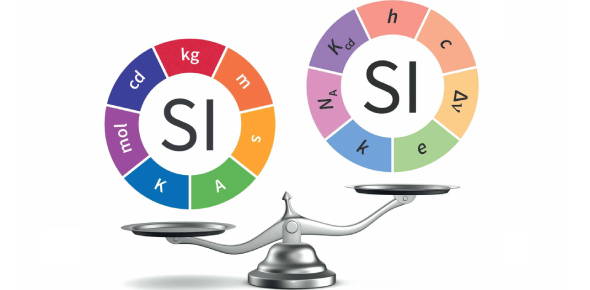# SI Units And Scientific Math Quiz! Trivia

Approved & Edited by ProProfs Editorial Team
At ProProfs Quizzes, our dedicated in-house team of experts takes pride in their work. With a sharp eye for detail, they meticulously review each quiz. This ensures that every quiz, taken by over 100 million users, meets our standards of accuracy, clarity, and engagement.
| Written by Jthompsn
J
Jthompsn
Community Contributor
Quizzes Created: 4 | Total Attempts: 683
Questions: 10 | Attempts: 243Settings.

• 1.

### Which of the following is NOT an SI unit?

• A.

Kilogram

• B.

Ounce

• C.

Meter

• D.

Second

B. Ounce
Explanation
The ounce is not an SI unit. The International System of Units (SI) is a globally recognized system for measurement, and it does not include the ounce. The kilogram, meter, and second are all SI units. The ounce is commonly used in the United States and some other countries for measuring weight, but it is not part of the SI system.

Rate this question:

• 2.

### Since area and volume are quantities that arise from other quantities (in this case--length), they are called

• A.

Derived quantities

• B.

Standard quantities

• C.

Metric quantities

• D.

Inaccurate quantities

A. Derived quantities
Explanation
Area and volume are derived quantities because they are calculated from other quantities, specifically length. They are not standard quantities or metric quantities, as those terms refer to different systems of measurement. Inaccurate quantities would be incorrect or imprecise measurements, which is not the case here. Therefore, the correct answer is derived quantities.

Rate this question:

• 3.

### Which of the following could NOT be used as a conversion factor?

• A.

1000mg/1g

• B.

1000km/1m

• C.

100cm/1m

• D.

10dL/1L

B. 1000km/1m
Explanation
A conversion factor is a ratio that expresses the relationship between two different units of measurement. It is used to convert between these units. In this question, all the given options can be used as conversion factors except for 1000km/1m. This is because the conversion factor should have the units in the numerator and denominator in a way that allows the units to cancel out. However, in the case of 1000km/1m, both units are in the numerator, which does not allow for proper cancellation of units.

Rate this question:

• 4.

### How many significant digits are in 0.003920057400?

• A.

8

• B.

10

• C.

12

• D.

13

B. 10
Explanation
The number 0.003920057400 has 10 significant digits. Significant digits are the digits that carry meaning in a number. In this case, all the digits from 3 to the last 0 are significant because they are not leading or trailing zeros. Therefore, the number has a total of 10 significant digits.

Rate this question:

• 5.

### 1 mL of water equals

• A.

1 g of water

• B.

0.001L of water

• C.

1 cm^3 of water

• D.

All of the other answers are equal to 1 mL of water

D. All of the other answers are equal to 1 mL of water
Explanation
The answer "all of the other answers are equal to 1 mL of water" is correct because 1 mL of water is equal to 1 g of water, 0.001 L of water, and 1 cm^3 of water. These units are all equivalent and can be used interchangeably when measuring the volume of water.

Rate this question:

• 6.

### A graph is titled "mass vs. volume". Which of the following could be units for the slope of the graph?

• A.

Cm^3/kg

• B.

G/cm^3

• C.

G/cm^2

• D.

ML/g

B. G/cm^3
Explanation
The slope of a graph represents the rate of change between the variables plotted on the x and y-axis. In this case, the graph is titled "mass vs. volume," so the slope would represent the change in mass per unit change in volume. The units for the slope can be determined by dividing the units of mass (g) by the units of volume (cm^3), resulting in g/cm^3. Therefore, g/cm^3 could be the units for the slope of the graph.

Rate this question:

• 7.

### The steps for showing your work on a laboratory calculation are

• A.

RERU

• B.

RESA

• C.

VFSA

• D.

VESU

C. VFSA
Explanation
The correct answer is VFSA. This stands for Verify, Formula, Substitute, and Answer. When showing your work on a laboratory calculation, you first verify the given information and the formula that needs to be used. Then, you substitute the values into the formula and perform the necessary calculations. Finally, you write down the answer with the correct units and any additional information required. This systematic approach ensures that the calculation is done accurately and allows others to follow your work.

Rate this question:

• 8.

### If a person finds a result of 15g/mL while the accepted is 20g/mL, this would represent a ____% error.

• A.

5

• B.

15

• C.

20

• D.

25

D. 25
Explanation
The person's result of 15g/mL is 25% lower than the accepted value of 20g/mL.

Rate this question:

• 9.

### The product of (3 x 10^2) cm  and (2 x 10^-10) cm  is

• A.

6 x 10^12 cm^2

• B.

6 x 10^-8 cm^2

• C.

6 x 10^8 cm^2

• D.

6 x 10^-12 cm^2

B. 6 x 10^-8 cm^2
Explanation
To multiply numbers in scientific notation, we multiply the coefficients and add the exponents. In this case, the coefficient is 6 (3 x 2) and the exponent is -8 (2 + (-10)). Therefore, the product of (3 x 10^2) cm and (2 x 10^-10) cm is 6 x 10^-8 cm^2.

Rate this question:

• 10.

### A graduated cylinder is more precise if it has

• A.

A smaller diameter (resulting in more markings)

• B.

A larger diameter

• C.

Great accuracy

• D.

No accuracyBack to top